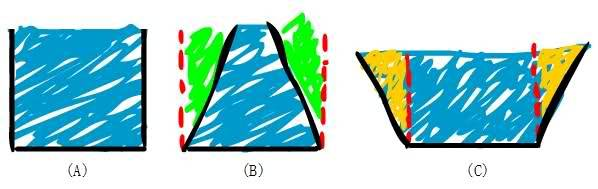# A Question About Weight and Pressure

Hello there :) First I'm sorry for my English it might be kinda bad !

I Have a little question about Weight and Pressure, Hope this is the right place.Here in this image we have 3 samples, A, B and C.

They are 3 different types of shapes fulled with a liquid (let's say water)

Talking about the physical pressure on the bottom of every shape :-

In Sample (A) we can see that Weight = Pressure.
In Sample (B) we can see that Weight < Pressure.
In Sample (C) we can see that Weight > Pressure.

Where did we get the extra pressure in the sample (B) ? It's like we create that green area from nowhere!
And where did the extra weight go in the sample (C) ? It's like we lost that orange area and it's nothing!

Hope I can fine the answer, Thank you :) !

## Answers and Replies

Doc Al
Mentor
In Sample (A) we can see that Weight = Pressure.
In Sample (B) we can see that Weight < Pressure.
In Sample (C) we can see that Weight > Pressure.
Where you say "weight", I assume you mean "weight/area". (Which would give you a pressure term.)
Where did we get the extra pressure in the sample (B) ? It's like we create that green area from nowhere.
The slanted walls exert a downward force.
And where did the extra weight go in the sample (C) ? It's like we lost that orange area, or it is nothing!
Here the slanted walls exert an upward force.

Delphi51
Homework Helper
Weight and pressure are very different things. For starters, weight is a force and has units of Newtons. Pressure is a force per unit area and has units of N/m². Weight only acts downward toward the center of the Earth. Pressure acts in all directions simultaneously.

Pressure is not like light intensity (energy/m²), either. If you increase the area on the bottom, the pressure at the bottom is spread over a larger area but it is not thereby diminished as light intensity would be if spread over a larger area. Consider a balloon. If you blow it up to a pressure of 1.1 atmospheres, then pump it up to 1.2 the balloon gets larger and the pressure is 1.2 on every bit of the larger surface area.

Where you say "weight", I assume you mean "weight/area". (Which would give you a pressure term.)

The slanted walls exert a downward force.

Here the slanted walls exert an upward force.

And where that forces come from ?!

Doc Al
Mentor
And where that forces come from ?!
The pressure of the water against the containing walls. Which, as pointed out by Delphi51, acts the same in all directions.

Doc Al and Delphi51 Thank you for your helping.
but when I explained it that way to my teacher he said that its not exactly the right answer.
I think he is looking for a really advance answer that explains it all.